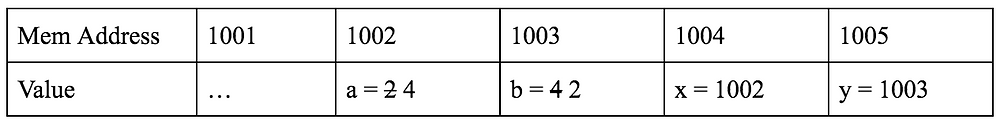# Pointers in C

### What is a pointer?

A pointer is simply a variable that stores the address of another variable.

An address of a variable is where the variable is located in memory. For example, if you declare int n = 8; in your program, then your computer’s memory would look something like this:From above, the address of the variable n is 1003.

If you want a variable that stores the address of a variable, not the value of a variable, then a pointer will be used

To declare a pointer, you simply append * (asterisk) next to a valid C data type and then a name of the pointer. The pointer will hold the address of a variable with the corresponding data type.

// a pointer to an int variable
int* int_ptr;

// a pointer to a double variable
double* double_ptr;

// a pointer to a char variable
char* char_ptr;

Let’s say you want to declare a pointer that stores the address of the variable n. To do this, you need to somehow access the address of n and assign it to a pointer. To access the address of a variable, you use & operator.

Consider the example below:

// assume the address of n is 1003
int n = 8;

// &n returns the address of n, which is 1003
int* int_ptr = &n;

In the example above, & operator is used to access the address of n, which is then assigned to a pointer int_ptr.

int_ptr stores the address of n. However, we might want to access the value at the address stored in a pointer. In other words, we might still want to access the value of n, using a pointer that stores the address of n. To do this, you use * operator.

Consider the example below:

// assume the address of n is 1003
int n = 8;

// &n returns the address of n, which is 1003
int* int_ptr = &n;

// value at address 1003 is 10, so val is 10
int val = *int_ptr;

In the example above, * operator is used to access the value at the address stored in int_ptr. The value at address 1003 is 10, so *int_ptr is 10. Using * operator on a pointer is called dereferencing a pointer.

### Why pointer?

At first, it can be confusing why we need to use pointers. Let’s consider a simple example that shows us how pointers can be useful.

void swap(int x, int y) {
int tmp = x;
x = y;
y = tmp;
}

int a = 2, b = 4;
printf("%d, %dn", a, b);
swap(a, b);
printf("%d, %dn", a, b);

The above is a simple program that calls swap() to swap the values of a and b. However, if you run this program, the values of a and b will not be changed. The reason is that when you pass a and b to swap(), the function makes its own copy of a and b to use. So, after the function ends, the original a and b will be unchanged. The swapping only happens within the function. The below table demonstrates this.void swap(int* x, int* y) {
int tmp = *x;
*x = *y;
*y = tmp;
}

int a = 2, b = 4;
printf("%d, %dn", a, b);
swap(&a, &b);
printf("%d, %dn", a, b);

The above is a correct program that uses pointers to swap the values of a and b. Notice how the program now passes the addresses of a and b to swap(), and the function’s parameters reflect this. In the body of the function, *x (the value at the address x) is assigned to tmp. Then, *y (the value at the address y) is assigned to *x (the value of the address x). Finally, tmp is assigned to *y (the value at the address y).

The latter program is correct because swap() uses the addresses of a and b to swap their values. So, after the function ends, a and b will be swapped because their addresses (and their corresponding values) were directly manipulated by the function. The below table demonstrates this.## Eunsub Lee

December 10, 2019

View Profile

#### Get in Touch

Thanks! We'll reach out shortly.